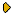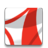Журнал Экспериментальной и Теоретической Физики
 НАЧАЛО | ПОИСК | ДЛЯ АВТОРОВ | ПОМОЩЬОбщая информация о журналеЗолотые страницыАдреса редакцииСодержание журналаСообщения редакцииПравила для авторовЗагрузить статьюПроверить статус статьиЖЭТФ, Том 149, Вып. 2, стр. 439 (Февраль 2016)
(Английский перевод - JETP, Vol. 122, No 2, February 2016 доступен on-line на www.springer.com )

Exact results on diffusion in a piecewise linear potential with a time-dependent sink

Поступила в редакцию: 31 Августа 2015

DOI: 10.7868/S0044451016020218PDF (154.5K)

The Smoluchowski equation with a time-dependent sink term is solved exactly. In this method, knowing the probability distribution P(0,s) at the origin, allows deriving the probability distribution P(x,s) at all positions. Exact solutions of the Smoluchowski equation are also provided in different cases where the sink term has linear, constant, inverse, and exponential variation in time.

Сообщить о технических проблемах